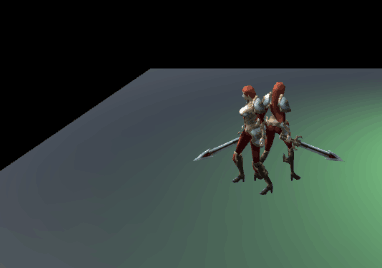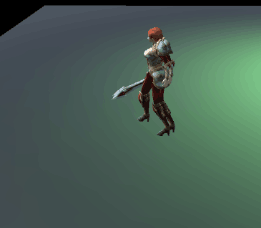unity3d游戏开发人物移动问题using System.Collections;
using System.Collections.Generic;
using UnityEngine;

public class playerMove : MonoBehaviour
{
public Transform myTransform;
private Ray ray;
private RaycastHit mHit;
//是否动作切换
private bool isCanAutoMove;
private Vector3 mTargetPos;

public Animator myAnimator;
private Camera _myCamera = null;
public float movespeed=5f;

void Start()
{
myTransform = gameObject.GetComponent ();
_myCamera = Camera.main;
myAnimator = GetComponent ();

}
void Update()
{
// 鼠标点击某个点，人物走向该地点
if (Input.GetMouseButton(0))
{
//返回一条射线 Ray从摄像机到屏幕指定的点
Ray ray = _myCamera.ScreenPointToRay (Input.mousePosition);
if (Physics.Raycast(ray, out mHit))
{
if (mHit.collider.gameObject.tag == "plane")
{
mTargetPos = mHit.point;
transform.LookAt(new Vector3(mTargetPos.x,mTargetPos.y,mTargetPos.z));
isCanAutoMove = true;
}
}
}
if (isCanAutoMove)
{

myAnimator.SetBool ("IdelOrRun",true);
transform.Translate(Vector3.forward * movespeed * Time.deltaTime);
myTransform.transform.Translate(Vector3.forward * movespeed * Time.deltaTime);
float distance = Vector3.Distance(mTargetPos, transform.position);
if (distance <= 0.5f)
{
isCanAutoMove = false;
myAnimator.SetBool ("IdelOrRun",false);
}
}
}
}

1个回答